## Example Questions

← Previous 1 3 4 5 6

### Example Question #1 : How To Find The Perimeter Of A Rhombus

If the area of a rhombus is 24 and one diagonal length is 6, find the perimeter of the rhombus.

8

12

20

16

24

20

Explanation:

The area of a rhombus is found by

A = 1/2(d1)(d2)

where d1 and d2 are the lengths of the diagonals.  Substituting for the given values yields

24 = 1/2(d1)(6)

24 = 3(d1)

8 = d1

Now, use the facts that diagonals are perpendicular in a rhombus, diagonals bisect each other in a rhombus, and the Pythagorean Theorem to determine that the two diagonals form 4 right triangles with leg lengths of 3 and 4.  Since 32 + 42 = 52, each side length is 5, so the perimeter is 5(4) = 20.

### Example Question #1 : Quadrilaterals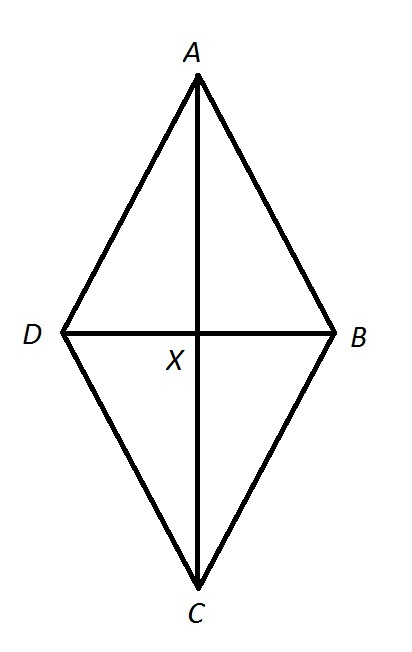Note: Figure NOT drawn to scale.

Calculate the perimeter of Quadrilateralin the above diagram if: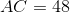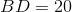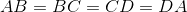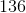Insufficient information is given to answer the question.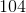Explanation:, so Quadrilateralis a rhombus. Its diagonals are therefore perpendicular to each other, and the four triangles they form are right triangles. Therefore, the Pythagorean theorem can be used to determine the common sidelength of Quadrilateral.

We focus on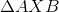. The diagonals are also each other's bisector, so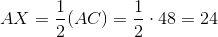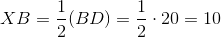By the Pythagorean Theorem,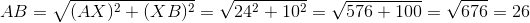26 is the common length of the four sides of Quadrilateral, so its perimeter is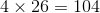.

### Example Question #242 : Geometry

A rhombus has a side length of 5. Which of the following is NOT a possible value for its area?

24

25

10

30

15

30

Explanation:

The area of a rhombus will vary as the angles made by its sides change. The "flatter" the rhombus is (with two very small angles and two very large angles, say 2, 178, 2, and 178 degrees), the smaller the area is. There is, of course, a lower bound of zero for the area, but the area can get arbitrarily small. This implies that the correct answer would be the largest choice. In fact, the largest area of a rhombus occurs when all four angles are equal, i.e. when the rhombus is a square. The area of a square of side length 5 is 25, so any value bigger than 25 is impossible to acheive.

### Example Question #2 : Quadrilaterals

In Rhombus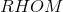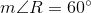. If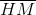is constructed, which of the following is true about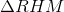?obtuse and scaleneis obtuse and isosceles, but not equilateralis acute and equilateralis acute and isosceles, but not equilateralis acute and scaleneis acute and equilateral

Explanation:

The figure referenced is below.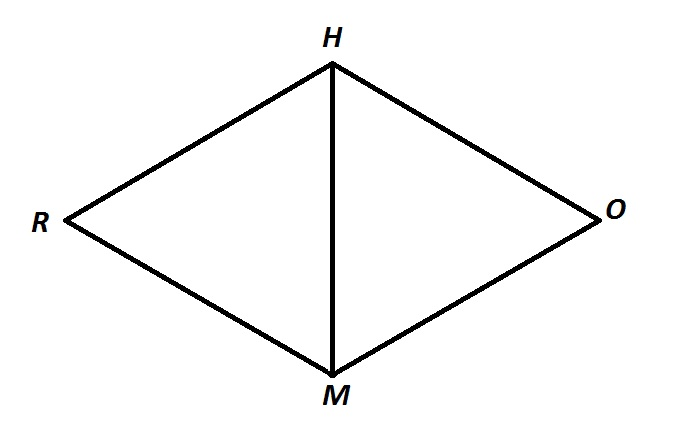Consecutive angles of a rhombus are supplementary - as they are with all parallelograms - so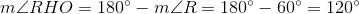A diagonal of a rhombus bisects its angles, so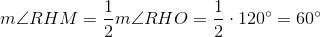A similar argument proves that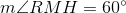.

Since all three angles ofmeasure, the triangle is acute. It is also equiangular, and, subsequently, equilateral.

### Example Question #1 : How To Find An Angle In A Rhombus

In Rhombus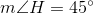. If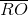is constructed, which of the following is true about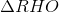?is right and scaleneis acute and scaleneis right and isosceles, but not equilateralis acute and isosceles, but not equilateralis acute and equilateralis acute and isosceles, but not equilateral

Explanation:

The figure referenced is below.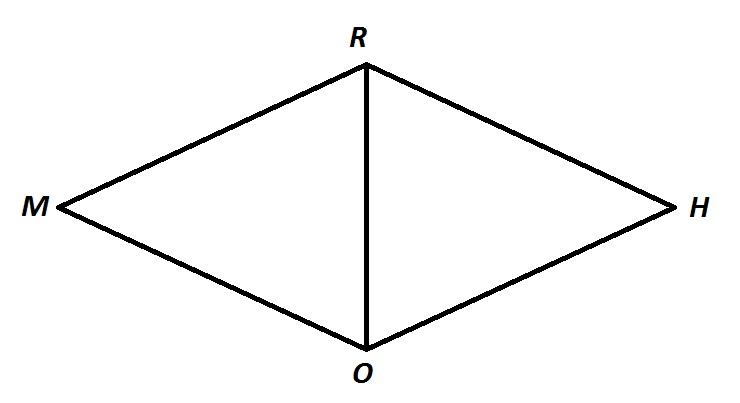The sides of a rhombus are congruent by definition, so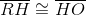, makingisosceles. It is not equilateral, since, and an equilateral triangle must have threeangles.

Also, consecutive angles of a rhombus are supplementary - as they are with all parallelograms - so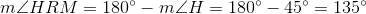A diagonal of a rhombus bisects its angles, so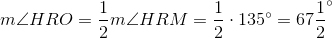Similarly,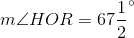This makesacute.

The correct response is thatis acute and isosceles, but not equilateral.

### Example Question #1 : Other Quadrilaterals

Quadrilateral ABCD contains four ninety-degree angles. Which of the following must be true?

I. Quadrilateral ABCD is a rectangle.

II. Quadrilateral ABCD is a rhombus.

III. Quadrilateral ABCD is a square.

II only

I and II only

II and III only

I, II, and III

I only

I only

Explanation:

Quadrilateral ABCD has four ninety-degree angles, which means that it has four right angles because every right angle measures ninety degrees. If a quadrilateral has four right angles, then it must be a rectangle by the definition of a rectangle. This means statement I is definitely true.

However, just because ABCD has four right angles doesn't mean that it is a rhombus. In order for a quadrilateral to be considered a rhombus, it must have four congruent sides. It's possible to have a rectangle whose sides are not all congruent. For example, if a rectangle has a width of 4 meters and a length of 8 meters, then not all of the sides of the rectangle would be congruent. In fact, in a rectangle, only opposite sides need be congruent. This means that ABCD is not necessarily a rhombus, and statement II does not have to be true.

A square is defined as a rhombus with four right angles. In a square, all of the sides must be congruent. In other words, a square is both a rectangle and a rhombus. However, we already established that ABCD doesn't have to be a rhombus. This means that ABCD need not be a square, because, as we said previously, not all of its sides must be congruent. Therefore, statement III isn't necessarily true either.

The only statement that has to be true is statement I.

### Example Question #9 : How To Find The Area Of A Trapezoid

A trapezoid has a base of length 4, another base of length s, and a height of length s. A square has sides of length s. What is the value of s such that the area of the trapezoid and the area of the square are equal?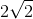Explanation:

In general, the formula for the area of a trapezoid is (1/2)(a + b)(h), where a and b are the lengths of the bases, and h is the length of the height. Thus, we can write the area for the trapezoid given in the problem as follows:

area of trapezoid = (1/2)(4 + s)(s)

Similarly, the area of a square with sides of length a is given by a2. Thus, the area of the square given in the problem is s2.

We now can set the area of the trapezoid equal to the area of the square and solve for s.

(1/2)(4 + s)(s) = s2

Multiply both sides by 2 to eliminate the 1/2.

(4 + s)(s) = 2s2

Distribute the s on the left.

4s + s2 = 2s2

Subtract s2 from both sides.

4s = s2

Because s must be a positive number, we can divide both sides by s.

4 = s

This means the value of s must be 4.

### Example Question #1 : How To Find The Area Of A Trapezoid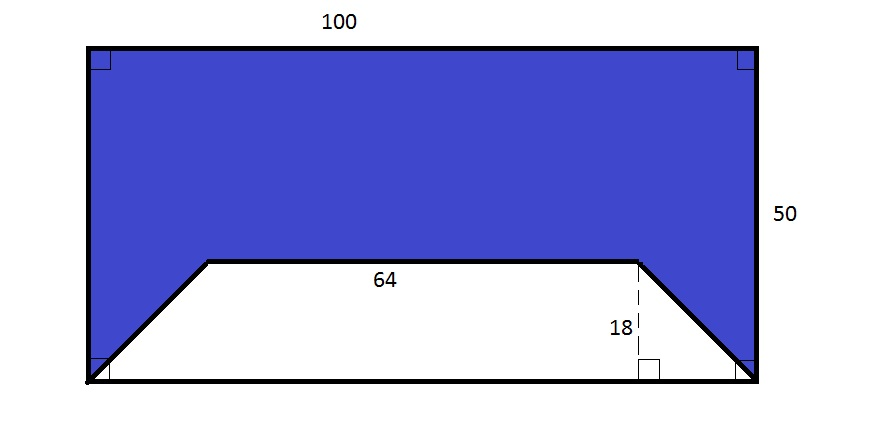Note: Figure NOT drawn to scale.

The white region in the above diagram is a trapezoid. What percent of the above rectangle, rounded to the nearest whole percent, is blue?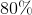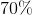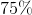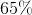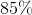Explanation:

The area of the entire rectangle is the product of its length and width, or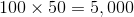.

The area of the white trapezoid is one half the product of its height and the sum of its base lengths, or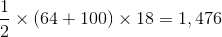Therefore, the blue polygon has area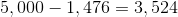This is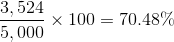of the rectangle.

Rounded, this is 70%.

### Example Question #2 : How To Find The Area Of A Trapezoid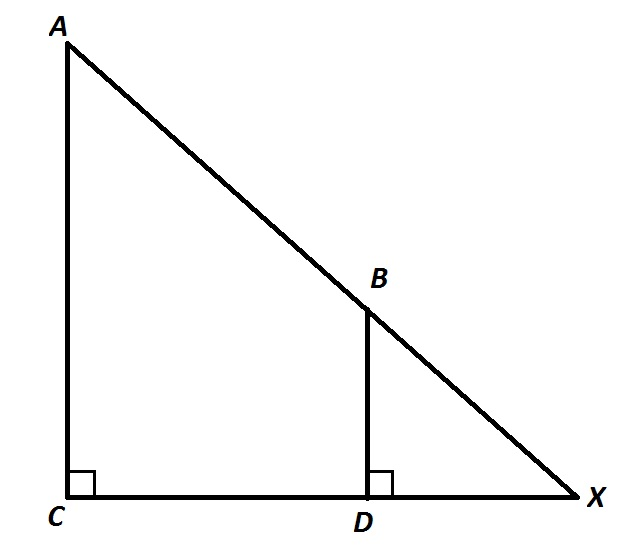Refer to the above diagram.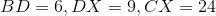.

Give the area of Quadrilateral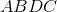.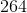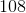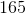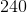Explanation: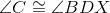, since both are right; by the Corresponding Angles Theorem,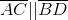, and Quadrilateralis a trapezoid.

By the Angle-Angle Similarity Postulate, sinceand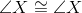(by reflexivity),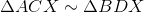and since corresponding sides of similar triangles are in proportion,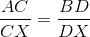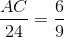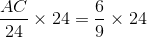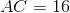, the larger base of the trapozoid;

The smaller base is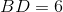.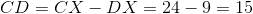, the height of the trapezoid.

The area of the trapezoid is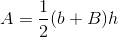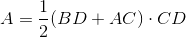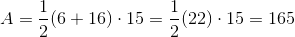### Example Question #5 : Quadrilaterals

A circle with a radius 2 in is inscribed in a square. What is the perimeter of the square?

32 in

16 in

24 in

12 in

28 in